# Random number generator within range in mql5116

Is it possible within MQL5 to generate a random number between a set starting point and ending point? Let's say I want a random number between 1000 and 2000. How would I do that?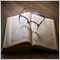6442

You can use this code:

```int  RandomNumber;

for(int i = 0; i< 1; i++) {
RandomNumber = MathRand();
if(RandomNumber > 2000 || RandomNumber < 1000) {
i--;
}
}
```

Have a nice day!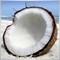4171

You can use interpolation to adjust result to required bounds:

```int MathRandomBounds(int minValue, int maxValue){
return minValue + MathRound((maxValue-minValue)*(MathRand()/32767.0));
}```

32767.0 is a constant maximal value for MathRand().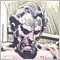3204

```#define RAND_MAX 32767
double MathRandomBounds(double minValue, double maxValue)
{
double f = (MathRand()/(double) RAND_MAX);
double retValue = minValue + f * (maxValue-minValue);
return retValue;
}```

I didn't see a clean way to use a template, i.e. template<typename T>, because of MathRound().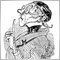Moderator
8149

hopokuk:
Is it possible within MQL5 to generate a random number between a set starting point and ending point? Let's say I want a random number between 1000 and 2000. How would I do that?

I would use just this:

` int rnd = 1000 + MathRand()%1000; // this gives 1000 - 1999`

(But the frequency of the numbers from 1000 to 1767 is a tiny tiny little bit higher than those from 1768-1999 but I guess it the fastest method.)1538

You can use this code to generate random numbers:

```//+------------------------------------------------------------------+
//| Random double in the range [min, max)                            |
//+------------------------------------------------------------------+
double MathRandDbl(const double min, const double max)
{
double f   = (MathRand() / 32768.0);
return min + (int)(f * (max - min));
}
//+------------------------------------------------------------------+
//| Random integer in the range [min, max)                           |
//| Generates random numbers with a uniform distribution (bias-free).|
//+------------------------------------------------------------------+
int MathRandInt(const int min, const int max)
{
int RAND_MAX = 32767;
int range = max - min;
if (range > RAND_MAX) range = RAND_MAX;
int randMin = RAND_MAX % range;
int rand;  do{ rand = MathRand(); }while (rand <= randMin);
return rand % range + min;
}
//+------------------------------------------------------------------+
//|                                                                  |
//+------------------------------------------------------------------+
void OnStart()
{
//--- Initialize the generator of random numbers
MathSrand(GetTickCount()^(uint)ChartID());

//--- Generate and print some random dates
for(int i=0; i<10; i++)
{
double rnd=MathRandDbl(D'1990.01.01',TimeCurrent());
Print((datetime)rnd);
}
//--- test disribution histogram
int hist[];
int bound=13;
int sample=100000;
ArrayResize(hist,bound);
ZeroMemory(hist);
for(int i=0; i<sample; i++)
hist[MathRandInt(0,bound)]++;
PrintFormat("histogram of MathRandInt(0,%d): ",bound);
ArrayPrint(hist,0,", ");
}
//+------------------------------------------------------------------+
```
Files: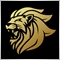Moderator
12380

Always remember that it is not cryptographically secure so don't use it to generate keys and wallets.1538

Marco vd Heijden:
Always remember that it is not cryptographically secure so don't use it to generate keys and wallets.

The above MathRandInt(function generates random integers in a uniform distribution (bias-free). It is enough to use it for general purposes.

Be aware that the following function commonly seen on this forum has a modulo bias. This can occur with some inputs when their range, b-a, is not dividing the whole range of the RNG evenly. It simply means that certain numbers are more likely to be drawn than others.

```//+------------------------------------------------------------------+
//| Modulo bias !!!                                                  |
//| https://stackoverflow.com/a/738651/360211                        |
//+------------------------------------------------------------------+
int MathRandInt(const int min, const int max)
{
double f   = (MathRand() / 32768.0);
return min + (int)(f * (max - min));
}
```

For more explanation on modulo bias, please refer to https://stackoverflow.com/a/738651/360211

Files:19155

So you eliminate the bias.
```// Returns a random number in the range [min..max) Max excluded.
// Random(10,  0)   returns 0   … 9      with equal probability.
// Random(10.0,0.0) returns 0.0 … 9.9999 with equal probability.
int RandomLinear(int max, int min=0){
int range   = max - min;
int randMax = 32768 - 32768 % range;
int rand;  do{ rand = MathRand(); }while (rand >= randMax);
return rand % range + min;
}```1538

William Roeder:
So you eliminate the bias.

Rounding to multiples of a step can be faster with:

`int randMax = (32768 / range) * range;`

You keep trying to get a random number in the max range divisible by b-a.

Also, you need to check that the range b-a is within RAND_MAX, otherwise we get an infinite loop here.

`int rand = RandomLinear(70123); `Moderator
17193

Why does this topic keep showing as having unread posts?

Is somebody constantly editing their post?